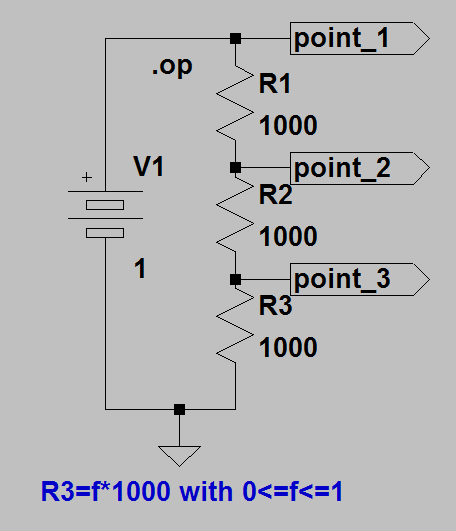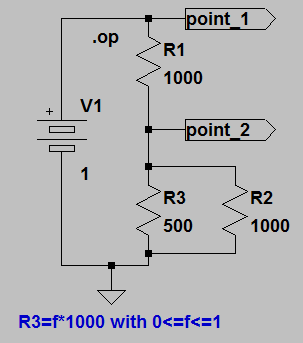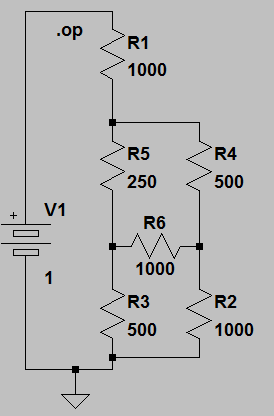## ECE 1810 (ENGRI 1810) Homework 1

1. The resistor R3 shown below varies from zero to 1 kohm. Write the value of the variable resistor as f*1000 where f varies between zero and one. Compute the voltage across each of the three resistors as a function of f. Check your answer by using LTspice to run this simulation with R3 = [200 500 1000]. Note that the simulation gives voltage with respect to ground at each point and you want voltages across each resistor, so you will need to subtract the results. For instance V(across R1) = V(point_1) - V(point_2).2. In the circuit shown below, the variable resistor R3 is defined as in problem 1. Compute the current through each resistor as a function of f. Check your answer by using LTspice to run a simulation with R3 = [200 500 1000].3. For the following circuit, use Kirchoff's laws to show that if R5/R3 = R4/R2, then the current through R6=0. You can check by simulation, but simulation by itself will not be considered a correct answer.August 30, 2011 Copyright Cornell University